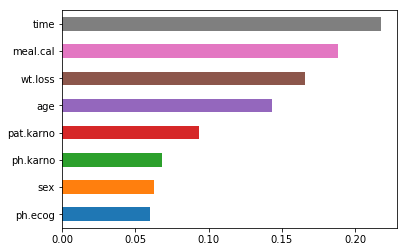# Random Forest in Python

This post will provide a demonstration of the use of the random forest algorithm in python. Random forest is similar to decision trees except that instead ofone tree a multitude of trees are grown to make predictions. The various trees all vote in terms of how to classify an example and majority vote is normally the winner. Through making many trees the accuracy of the model normally improves.

The steps are as follows for the use of random forest

1. Data preparation
2. Model development & evaluation
3. Model comparison
4. Determine variable importance

Data Preparation

We will use the cancer dataset from the pydataset module. We want to predict if someone is censored or dead in the status variable. The other variables will be used as predictors. Below is some code that contains all of the modules we will use.

```import pandas as pd
import sklearn.ensemble as sk
from pydataset import data
from sklearn.model_selection import train_test_split
from sklearn import metrics
import matplotlib.pyplot as plt```

We will now load our data cancer in an object called ‘df’. Then we will remove all NA’s use the .dropna() function. Below is the code.

```df = data('cancer')
df=df.dropna()```

We now need to make two datasets. One dataset, called X, will contain all of the predictor variables. Another dataset, called y, will contain the outcome variable. In the y dataset, we need to change the numerical values to a string. This will make interpretation easier as we will not need to lookup what the numbers represents. Below is the code.

```X=df[['time','age',"sex","ph.ecog",'ph.karno','pat.karno','meal.cal','wt.loss']]
df['status']=df.status.replace(1,'censored')
y=df['status']```

Instead of 1 we now have the string “censored” and instead of 2 we now have the string “dead” in the status variable. The final step is to set up our train and test sets. We will do a 70/30 split. We will have a train set for the X and y dataset as well as a test set for the X and y datasets. This means we will have four datasets in all. Below is the code.

`x_train, x_test, y_train, y_test = train_test_split(X, y, test_size=0.3, random_state=0)`

We are now ready to move to model development

Model Development and Evaluation

We now need to create our classifier and fit the data to it. This is done with the following code.

```clf=sk.RandomForestClassifier(n_estimators=100)
clf=clf.fit(x_train,y_train)```

The clf object has our random forest algorithm,. The number of estimators is set to 100. This is the number of trees that will be generated. In the second line of code, we use the .fit function and use the training datasets x and y.

We now will test our model and evaluate it. To do this we will use the .predict() with the test dataset Then we will make a confusion matrix followed by common metrics in classification. Below is the code and the output.You can see that our model is good at predicting who is dead but struggles with predicting who is censored. The metrics are reasonable for dead but terrible for censored.

We will now make a second model for the purpose of comparison

Model Comparison

We will now make a different model for the purpose of comparison. In this model, we will use out of bag samples to determine accuracy, set the minimum split size at 5 examples, and that each leaf has at least 2 examples. Below is the code and the output.There was some improvement in classify people who were censored as well as for those who were dead.

Variable Importance

We will now look at which variables were most important in classifying our examples. Below is the code

```model_ranks=pd.Series(clf.feature_importances_,index=x_train.columns,name="Importance").sort_values(ascending=True,inplace=False)
ax=model_ranks.plot(kind='barh')```

We create an object called model_ranks and we indicate the following.

1. Classify the features by importance
2. Set index to the columns in the training dataset of x
3. Sort the features from most to least importance
4. Make a barplot

Below is the outputYou can see that time is the strongest classifier. How long someone has cancer is the strongest predictor of whether they are censored or dead. Next is the number of calories per meal followed by weight and lost and age.

Conclusion

Here we learned how to use random forest in Python. This is another tool commonly used in the context of machine learning.